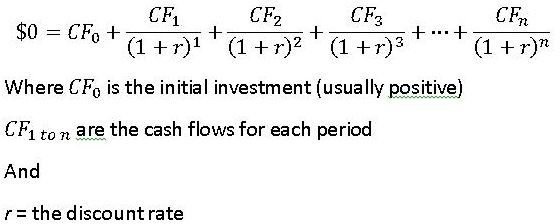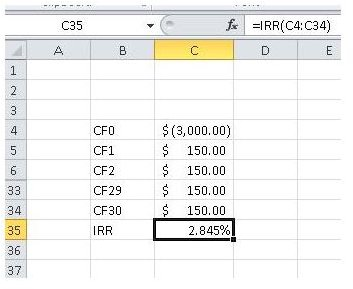# Learn How to Calculate IRR in Excel for Monthly and Annualized Cash Flow

Page content

The internal rate of return (IRR) for a project can be defined as the discount rate that offers zero net present value (NPV) or the rate where the present value of the initial investment or cash outflows is the same amount as the present value of the future cash inflows that are associated with a project. We will discuss how to calculate IRR in Excel for determining your monthly cash flow.Project budgets are a critical component Calculating the IRR for a series of cash flows requires a basic formula that looks something like the one in the image below (click to enlarge).IRR equation

Notice that the IRR formula does not define the period for each cash flow. This means that the IRR can be calculated for a year, a month, a week, or even a day as long as the person performing the calculations remembers what period was used in each calculation. In the case a monthly IRR is calculated, the period could be a week, a day, or any other defined period in hours or minutes, depending on the nature of the cash flows that are involved. IRR is used to make budgeting decisions when deciding which (if any) projects are to be adopted. Many organizations have a required rate of return that projects must meet in order to be funded. If a project is shown to exceed that rate of return (also called the hurdle rate), the project can be considered for funding. Any project that fails to exceed the hurdle rate is normally declined.

## Calculating Monthly IRR

Before a manager can convert monthly IRR to annual IRR, the monthly IRR should be determined. This can be most easily done based on daily or weekly cash flows, but could be calculated based on any defined period. Remembering what periods are used can be helpful at arriving at an accurate IRR calculation. Because the IRR calculation makes solving for the discount rate a difficult task, consider using a spreadsheet such as Microsoft Excel to handle the calculations. It has a built in function called “IRR” that will accurately compute the internal rate of return for a series of cash flows. As an example, let’s suppose a company spent \$3,000 to get a car for a month for a short term project of renting them out to customers. Daily regular net cash inflows from the car could be \$150 per day. Assuming 30 days in the month, a monthly IRR could be calculated as shown in the image below (click to enlarge).Excel has a built-in IRR function!

Using the formula, =IRR(C4:C34), the monthly IRR from daily net cash inflows is 2.845%, but what is that in terms of an annual rate?

## Annualizing a Monthly IRR

With a monthly number to work with, a manager can convert monthly IRR to annual IRR. This can be helpful to put the monthly return in perspective or to form a comparison with another project that has an annualized IRR. When annualizing a return, use the following formula to account for compounding (simply multiplying the monthly rate by twelve won’t work): (1+r)12 -1. Punching in the monthly IRR into the equation yields, (1+.02845)12-1 = .40023, or 40.023%.

## Summary

Internal rate of return has been defined, explained, and applied to a daily net inflow of cash over the course of a month. We showed you how to calculate IRR in Excel and after that, the way to convert monthly IRR to annual IRR was explained and demonstrated. The examples provided here assume that cash flows are regular. Screenshots taken by Bruce Tyson Budget Image by Steve Buissinne from Pixabay